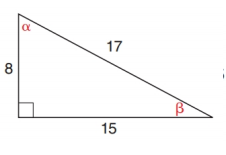Chapter 11.2, Problem 2EElementary Geometry For College St...

7th Edition
Alexander + 2 others
ISBN: 9781337614085

Solutions

Chapter
SectionElementary Geometry For College St...

7th Edition
Alexander + 2 others
ISBN: 9781337614085
Textbook Problem

In Exercises 1 to 6, find cos α and cos β .To determine

To find:

cosα and cosβ.

Explanation

Consider the following figure,

General formula for cosine ratio is given below,

From the given figure the value 17 is the hypotenuse value since it is opposite to right angle.

Then 8 is the length of the leg adjacent to α and 15 is the length of leg adjacent to β

Still sussing out bartleby?

Check out a sample textbook solution.

See a sample solution

The Solution to Your Study Problems

Bartleby provides explanations to thousands of textbook problems written by our experts, many with advanced degrees!

Get Started

Find more solutions based on key concepts